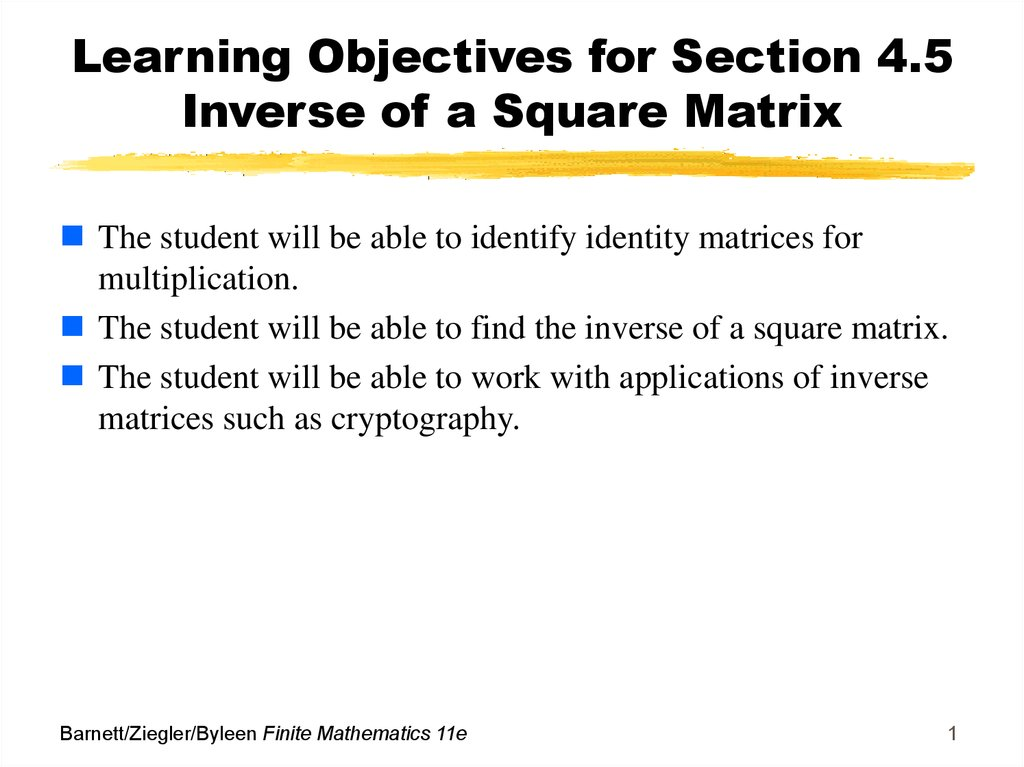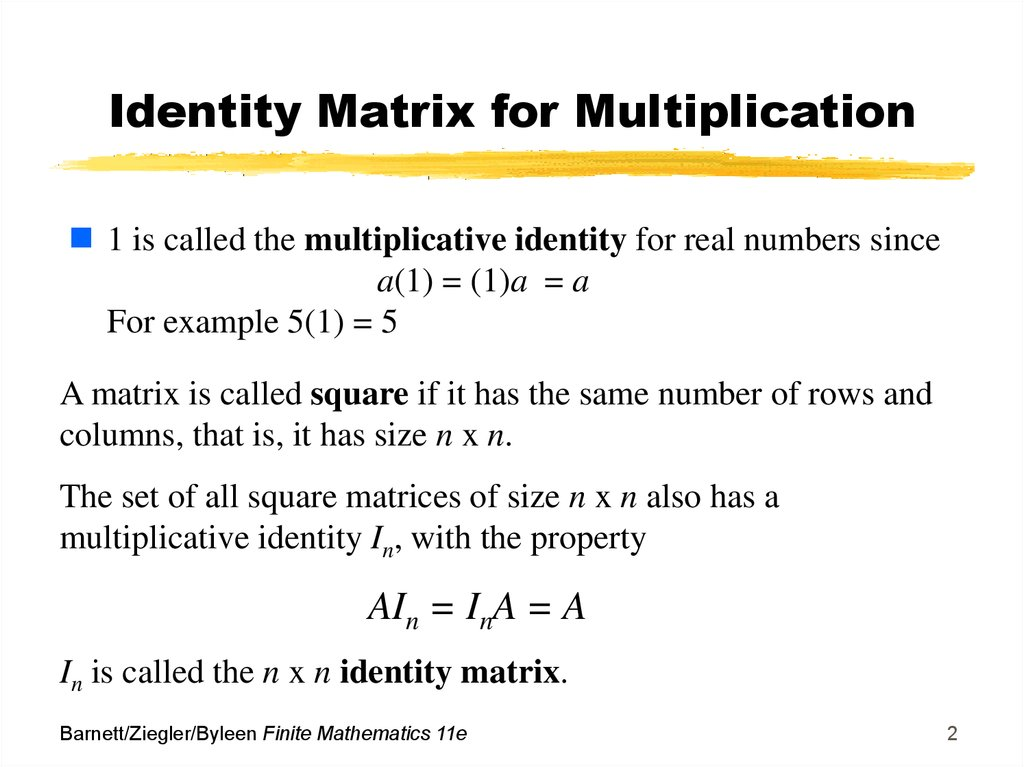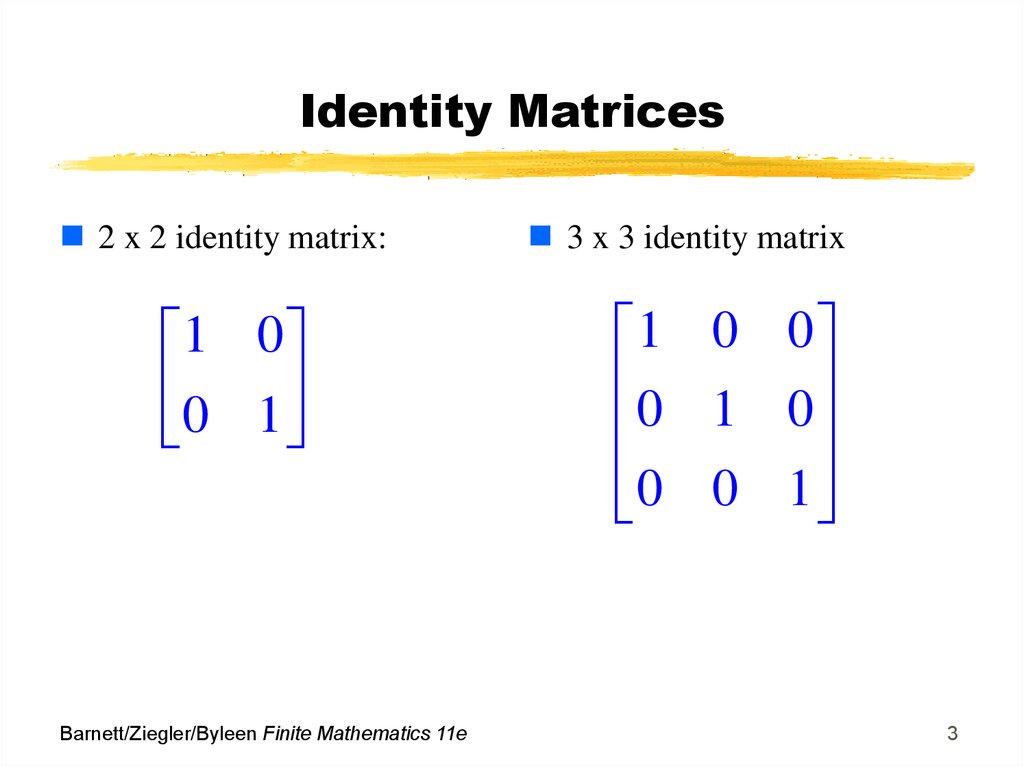# Inverse of a Square Matrix

## 1. Learning Objectives for Section 4.5 Inverse of a Square Matrix

The student will be able to identify identity matrices for
multiplication.
The student will be able to find the inverse of a square matrix.
The student will be able to work with applications of inverse
matrices such as cryptography.
Barnett/Ziegler/Byleen Finite Mathematics 11e
1

## 2. Identity Matrix for Multiplication

1 is called the multiplicative identity for real numbers since
a(1) = (1)a = a
For example 5(1) = 5
A matrix is called square if it has the same number of rows and
columns, that is, it has size n x n.
The set of all square matrices of size n x n also has a
multiplicative identity In, with the property
AIn = InA = A
In is called the n x n identity matrix.
Barnett/Ziegler/Byleen Finite Mathematics 11e
2

## 3. Identity Matrices

2 x 2 identity matrix:
1 0
0 1
Barnett/Ziegler/Byleen Finite Mathematics 11e
3 x 3 identity matrix
1 0 0
0 1 0
0 0 1
3

## 4. Identity Matrix Multiplication

AI = A (Verify the multiplication)
We can also show that IA = A and in general AI = IA = A for
all square matrices A.
a11 a12 a13 1 0 0 a11 a12 a13
a
a
a
0
1
0
a
a
a
21
22
23
21
22
23
a a a 0 0 1 a a a
31 32 33
31 32 33
Barnett/Ziegler/Byleen Finite Mathematics 11e
4

## 5. Inverse of a Matrix

All real numbers (excluding 0) have an inverse.
1
a 1
a
.
For example
1
5 1
5
Barnett/Ziegler/Byleen Finite Mathematics 11e
5

## 6. Matrix Inverses

Some (not all) square matrices also have matrix inverses
If the inverse of a matrix A exists, we shall call it A-1
Then
1
1
A A A A In
Barnett/Ziegler/Byleen Finite Mathematics 11e
6

## 7. Inverse of a 2x2 Matrix

There is a simple procedure to find the inverse of a two by
two matrix. This procedure only works for the 2 x 2 case.
An example will be used to illustrate the procedure.
Example: Find the inverse of
2 3
1 2
Barnett/Ziegler/Byleen Finite Mathematics 11e
7

## 8. Inverse of a 2x2 matrix (continued)

Step 1: Determine whether or not the inverse actually exists.
We define Δ = the difference of the product of the diagonal
elements of the matrix.
2 . 3
1 2
In order for the inverse of a 2 x 2 matrix to exist, Δ cannot
equal zero.
If Δ happens to be zero, then we conclude the inverse does
not exist, and we stop all calculations.
In our case Δ = 2(2)-1(3) = 1, so we can proceed.
Barnett/Ziegler/Byleen Finite Mathematics 11e
8

## 9. Inverse of a 2x2 matrix (continued)

2 3
1 2
2 3
1 2
Barnett/Ziegler/Byleen Finite Mathematics 11e
Step 2. Reverse the entries on
the main diagonal.
In this example, both entries
are 2, and no change is visible.
Step 3. Reverse the signs of
the other diagonal entries 3 and
1 so they become -3 and -1
Step 4. Divide each element
of the matrix by which in this
case is 1, so no apparent
change will be noticed.
9

## 10. Inverse of a 2x2 matrix (continued)

The inverse of the matrix is then
2 3
1 2
To verify that this is the inverse, we will multiply the original
matrix by its inverse and hopefully obtain the 2 x 2 identity
matrix:
2 3 2 3
1 2 1 2
Barnett/Ziegler/Byleen Finite Mathematics 11e
=
4 3 6 6 1 0
2 2 3 4 0 1
10

## 11. Inverse of a General Square Matrix

1. Augment the matrix with the n x n identity matrix.
2. Use elementary row operations to transform the matrix on the
left side of the vertical line to the n x n identity matrix. The
row operations are used for the entire row, so that the matrix
on the right hand side of the vertical line will also change.
3. When the matrix on the left is transformed to the n x n identity
matrix, the matrix on the right of the vertical line is the
inverse.
Barnett/Ziegler/Byleen Finite Mathematics 11e
11

## 12. Example: Inverse of a 3x3 Matrix

Find the inverse of
1 1 3
2 1 2
2 2 1
Step 1. Multiply R1 by (-2) and add the result to R2.
Step 2. Multiply R1 by 2 and add the result to R3
Barnett/Ziegler/Byleen Finite Mathematics 11e
12

## 13. Example (continued)

Step 3. Multiply row 2 by (1/3) to get a 1 in the second
row, first position.
Step 4. Add R2 to R1.
Step 5. Multiply R2 by 4 and add the result to R3.
Step 6. Multiply R3 by 3/5 to get a 1 in the third row, third
position.
Barnett/Ziegler/Byleen Finite Mathematics 11e
13

## 14. Example (continued)

Step 7. Eliminate the (5/3) in the first row, third position by
multiplying R3 by (-5/3) and adding result to R1.
Step 8. Eliminate the (-4/3) in the second row, third position
by multiplying R3 by (4/3) and adding result to R2.
Step 9. You now have the identity matrix on the left, which
is our goal.
Barnett/Ziegler/Byleen Finite Mathematics 11e
14

## 15. Example Solution

The inverse matrix appears on the right hand side of the
vertical line and is displayed below. Many calculators as well
as computers have software programs that can calculate the
inverse of a matrix quite easily. If you have access to a TI 83,
consult the manual to determine how to find the inverse using
a calculator.
Barnett/Ziegler/Byleen Finite Mathematics 11e
15

## 16. Application: Cryptography

Matrix inverses can provide a simple and effective procedure for
encoding and decoding messages. To begin, assign the numbers
1-26 to the letters in the alphabet, as shown below. Also assign
the number 0 to a blank to provide for space between words.
Blank A B C D E F G H I J K L M N O P Q R S T U V W X Y Z
0
1 2 3 4 5 6 7 8 9 10 11 12 13 14 15 16 17 18 19 20 21 22 23 24 25 26
Thus the message “SECRET CODE” corresponds to the sequence
19 5 3 18 5 20 0 3 15 4 5
Barnett/Ziegler/Byleen Finite Mathematics 11e
16

## 17. Cryptography (continued)

Any matrix A whose elements are positive integers and
whose inverse exists can be used as an encoding matrix.
For example, to use the 2 x 2 matrix
4 3
A
1
1
to encode the message above, first divide the numbers in
the sequence 19 5 3 18 5 20 0 3 15 4 5 into groups
of 2, and use these groups as the columns of a matrix B:
19 3 5 0 15 5
B
5
18
20
3
4
0
We added an extra blank at the end of the message to
make the columns come out even.
Barnett/Ziegler/Byleen Finite Mathematics 11e
17

## 18. Cryptography (continued)

Then we multiply this matrix on the left by A:
4 3 19 3 5 0 15 5
AB
5 18 20 3 4 0
1
1
91 66 80 9 72 20
24
21
25
3
19
5
The coded message is
91 24 66 21 80 25 9 3 72 19 20 5
This message can be decoded simply by putting it back into matrix
form and multiplying on the left by the decoding matrix A-1.
Barnett/Ziegler/Byleen Finite Mathematics 11e
18